# Uncertainty Chapter 13 Outline Uncertainty Probability Syntax and

• Slides: 23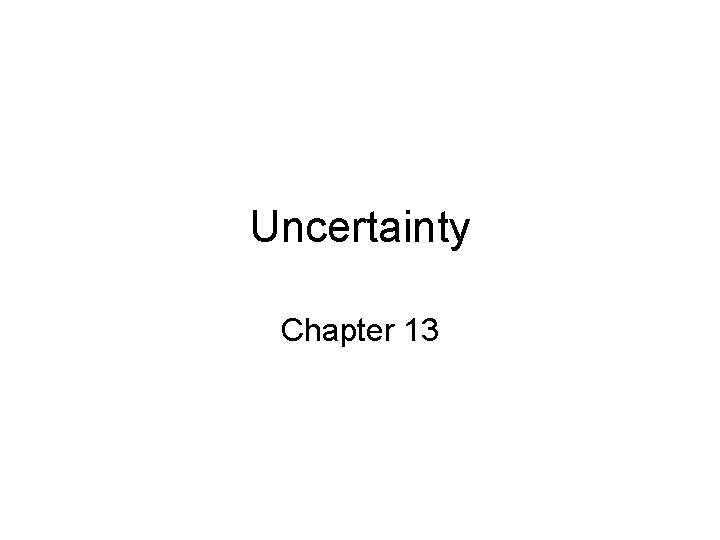Uncertainty Chapter 13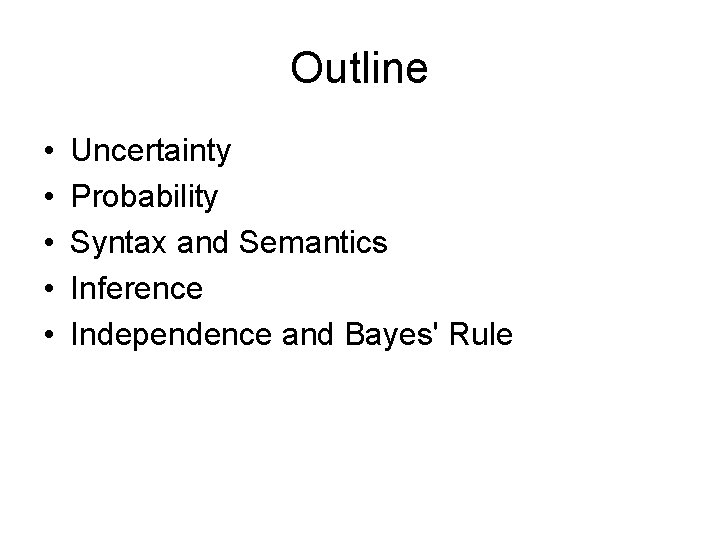Outline • • • Uncertainty Probability Syntax and Semantics Inference Independence and Bayes' Rule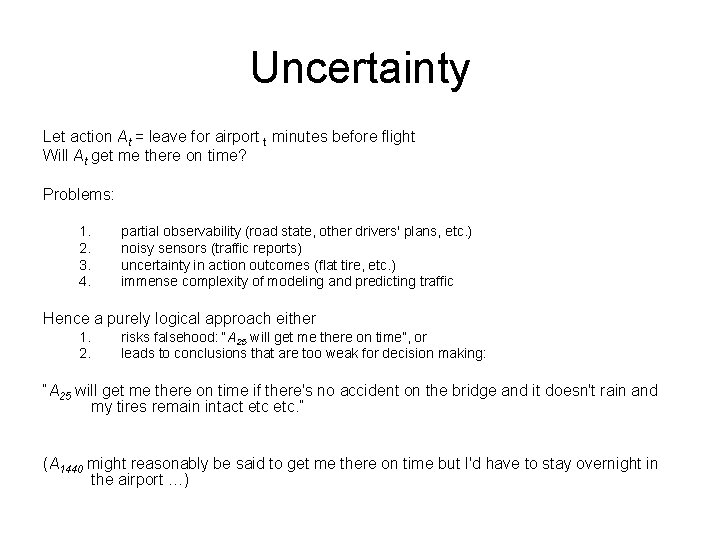Uncertainty Let action At = leave for airport t minutes before flight Will At get me there on time? Problems: 1. 2. 3. 4. partial observability (road state, other drivers' plans, etc. ) noisy sensors (traffic reports) uncertainty in action outcomes (flat tire, etc. ) immense complexity of modeling and predicting traffic Hence a purely logical approach either 1. 2. risks falsehood: “A 25 will get me there on time”, or leads to conclusions that are too weak for decision making: “A 25 will get me there on time if there's no accident on the bridge and it doesn't rain and my tires remain intact etc. ” (A 1440 might reasonably be said to get me there on time but I'd have to stay overnight in the airport …)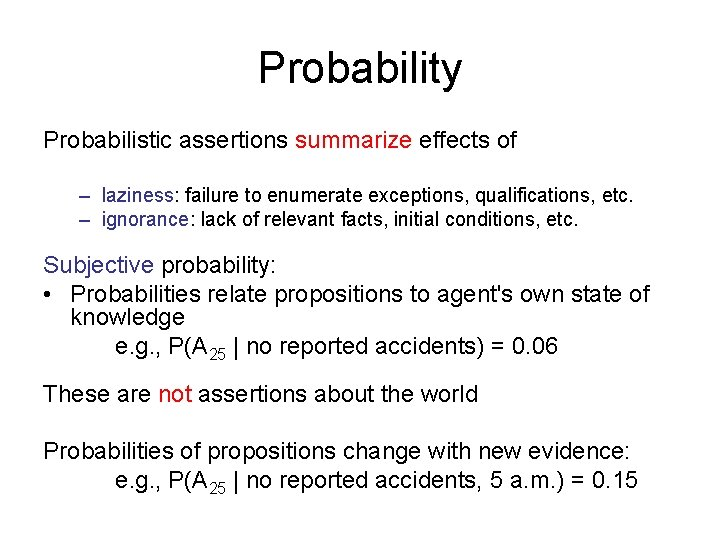Probability Probabilistic assertions summarize effects of – laziness: failure to enumerate exceptions, qualifications, etc. – ignorance: lack of relevant facts, initial conditions, etc. Subjective probability: • Probabilities relate propositions to agent's own state of knowledge e. g. , P(A 25 | no reported accidents) = 0. 06 These are not assertions about the world Probabilities of propositions change with new evidence: e. g. , P(A 25 | no reported accidents, 5 a. m. ) = 0. 15Making decisions under uncertainty Suppose I believe the following: P(A 25 gets me there on time | …) P(A 90 gets me there on time | …) P(A 120 gets me there on time | …) P(A 1440 gets me there on time | …) = 0. 04 = 0. 70 = 0. 95 = 0. 9999 • Which action to choose? Depends on my preferences for missing flight vs. time spent waiting, etc. – Utility theory is used to represent and infer preferences – Decision theory = probability theory + utility theorySyntax • • • Basic element: random variable Similar to propositional logic: possible worlds defined by assignment of values to random variables. Boolean random variables e. g. , Cavity (do I have a cavity? ) • Discrete random variables e. g. , Weather is one of <sunny, rainy, cloudy, snow> • Domain values must be exhaustive and mutually exclusive • Elementary proposition constructed by assignment of a value to a random variable: e. g. , Weather = sunny, Cavity = false (abbreviated as cavity) • • Complex propositions formed from elementary propositions and standard logical connectives e. g. , Weather = sunny Cavity = false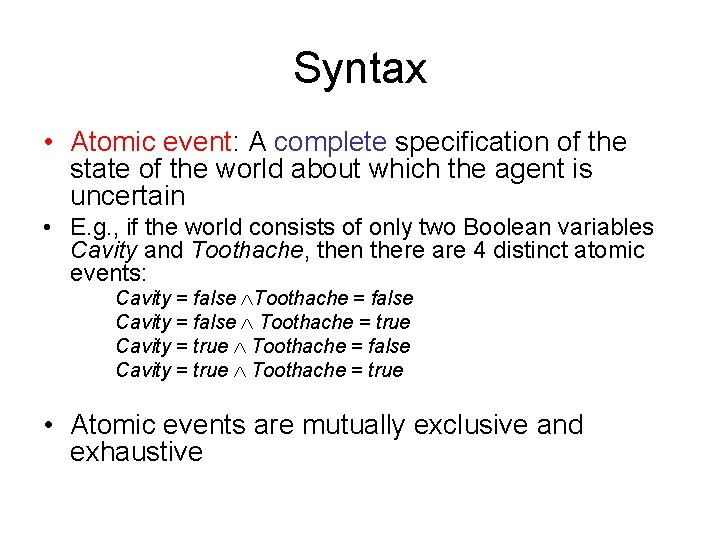Syntax • Atomic event: A complete specification of the state of the world about which the agent is uncertain • E. g. , if the world consists of only two Boolean variables Cavity and Toothache, then there are 4 distinct atomic events: Cavity = false Toothache = false Cavity = false Toothache = true Cavity = true Toothache = false Cavity = true Toothache = true • Atomic events are mutually exclusive and exhaustiveAxioms of probability • For any propositions A, B – 0 ≤ P(A) ≤ 1 – P(true) = 1 and P(false) = 0 – P(A B) = P(A) + P(B) - P(A B)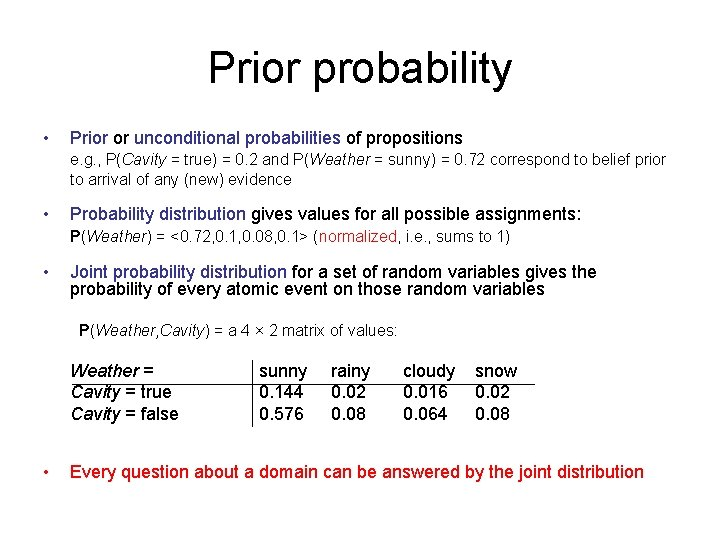Prior probability • Prior or unconditional probabilities of propositions e. g. , P(Cavity = true) = 0. 2 and P(Weather = sunny) = 0. 72 correspond to belief prior to arrival of any (new) evidence • Probability distribution gives values for all possible assignments: P(Weather) = <0. 72, 0. 1, 0. 08, 0. 1> (normalized, i. e. , sums to 1) • Joint probability distribution for a set of random variables gives the probability of every atomic event on those random variables P(Weather, Cavity) = a 4 × 2 matrix of values: Weather = Cavity = true Cavity = false • sunny 0. 144 0. 576 rainy 0. 02 0. 08 cloudy 0. 016 0. 064 snow 0. 02 0. 08 Every question about a domain can be answered by the joint distributionConditional probability • Conditional or posterior probabilities • e. g. , P(cavity | toothache) = 0. 8 • (Notation for conditional distributions: • P(Cavity | Toothache) = 2 -element vector of 2 -element vectors) • If we know more, e. g. , cavity is also given, then we have • P(cavity | toothache, cavity) = 1 • New evidence may be irrelevant, allowing simplification, e. g. , • P(cavity | toothache, sunny) = P(cavity | toothache) = 0. 8 • This kind of inference, sanctioned by domain knowledge, is crucialConditional probability • Definition of conditional probability: • P(a | b) = P(a b) / P(b) if P(b) > 0 • Product rule gives an alternative formulation: • P(a b) = P(a | b) P(b) = P(b | a) P(a) • A general version holds for whole distributions, e. g. , • P(Weather, Cavity) = P(Weather | Cavity) P(Cavity) • Chain rule is derived by successive application of product rule: P(X 1, …, Xn) = P(X 1, . . . , Xn-1) P(Xn | X 1, . . . , Xn-1) = P(X 1, . . . , Xn-2) P(Xn-1 | X 1, . . . , Xn-2) P(Xn | X 1, . . . , Xn-1) =… = πi= 1^n P(Xi | X 1, … , Xi-1)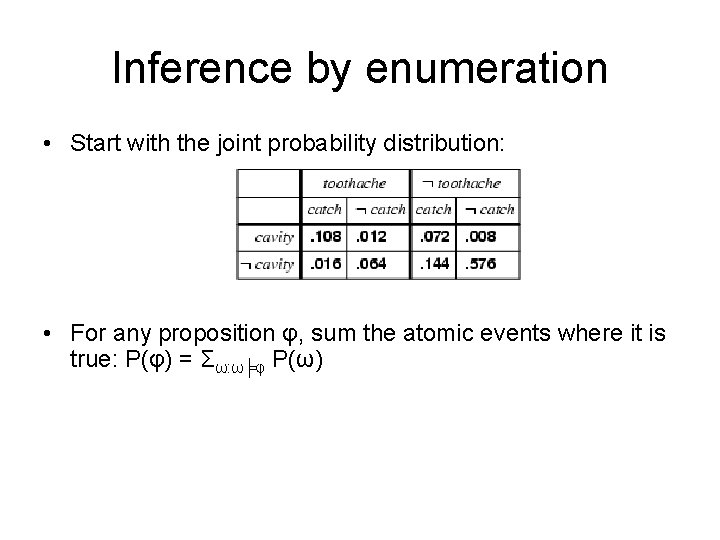Inference by enumeration • Start with the joint probability distribution: • For any proposition φ, sum the atomic events where it is true: P(φ) = Σω: ω╞φ P(ω)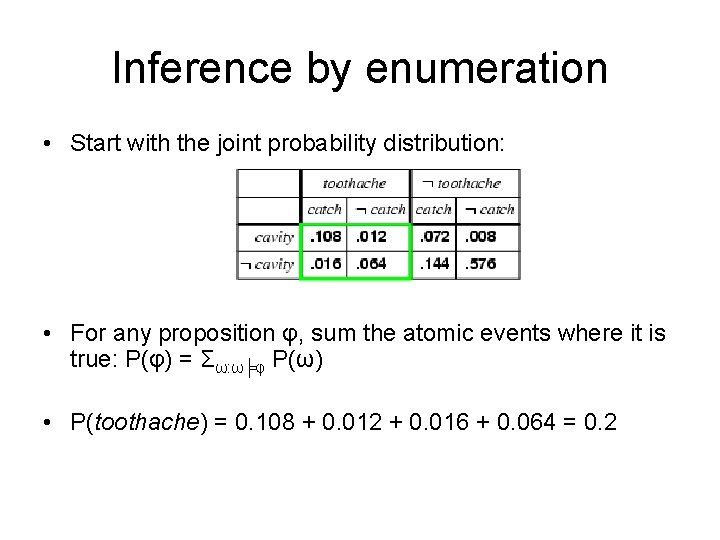Inference by enumeration • Start with the joint probability distribution: • For any proposition φ, sum the atomic events where it is true: P(φ) = Σω: ω╞φ P(ω) • P(toothache) = 0. 108 + 0. 012 + 0. 016 + 0. 064 = 0. 2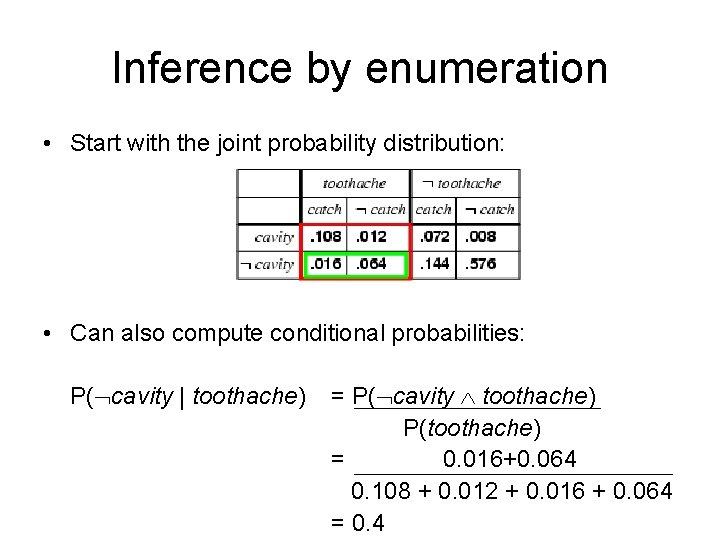Inference by enumeration • Start with the joint probability distribution: • Can also compute conditional probabilities: P( cavity | toothache) = P( cavity toothache) P(toothache) = 0. 016+0. 064 0. 108 + 0. 012 + 0. 016 + 0. 064 = 0. 4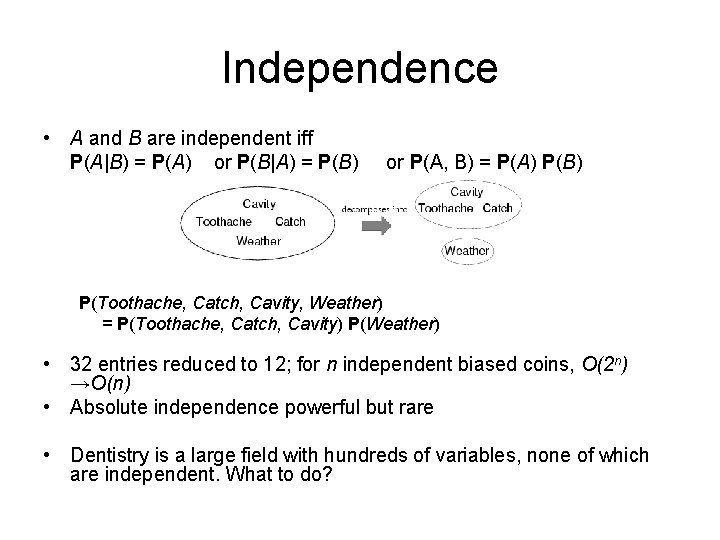Independence • A and B are independent iff P(A|B) = P(A) or P(B|A) = P(B) or P(A, B) = P(A) P(B) P(Toothache, Catch, Cavity, Weather) = P(Toothache, Catch, Cavity) P(Weather) • 32 entries reduced to 12; for n independent biased coins, O(2 n) →O(n) • Absolute independence powerful but rare • Dentistry is a large field with hundreds of variables, none of which are independent. What to do?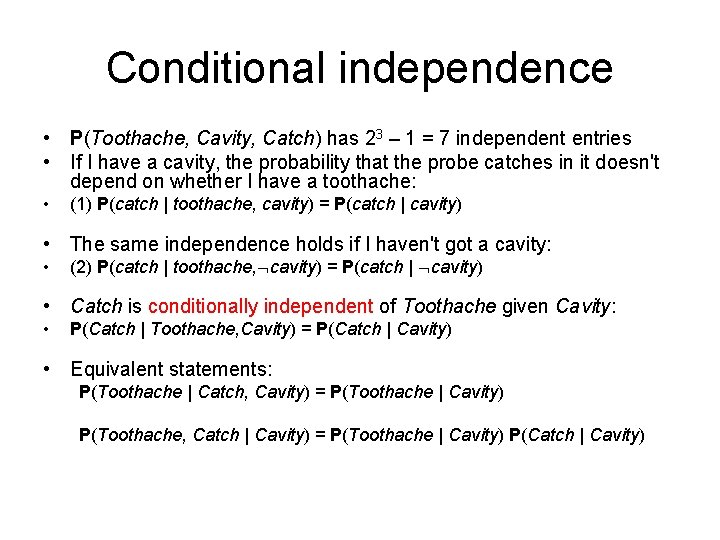Conditional independence • P(Toothache, Cavity, Catch) has 23 – 1 = 7 independent entries • If I have a cavity, the probability that the probe catches in it doesn't depend on whether I have a toothache: • (1) P(catch | toothache, cavity) = P(catch | cavity) • The same independence holds if I haven't got a cavity: • (2) P(catch | toothache, cavity) = P(catch | cavity) • Catch is conditionally independent of Toothache given Cavity: • P(Catch | Toothache, Cavity) = P(Catch | Cavity) • Equivalent statements: P(Toothache | Catch, Cavity) = P(Toothache | Cavity) P(Toothache, Catch | Cavity) = P(Toothache | Cavity) P(Catch | Cavity)Conditional independence contd. • Write out full joint distribution using chain rule: P(Toothache, Catch, Cavity) = P(Toothache | Catch, Cavity) P(Catch | Cavity) P(Cavity) = P(Toothache | Cavity) P(Catch | Cavity) P(Cavity) I. e. , 2 + 1 = 5 independent numbers • In most cases, the use of conditional independence reduces the size of the representation of the joint distribution from exponential in n to linear in n.Bayes' Rule • Product rule P(a b) = P(a | b) P(b) = P(b | a) P(a) • Bayes' rule: P(a | b) = P(b | a) P(a) / P(b) • or in distribution form • P(Y|X) = P(X|Y) P(Y) / P(X) = αP(X|Y) P(Y) • Useful for assessing diagnostic probability from causal probability: – P(Cause|Effect) = P(Effect|Cause) P(Cause) / P(Effect) – E. g. , let M be meningitis, S be stiff neck: P(m|s) = P(s|m) P(m) / P(s) = 0. 8 × 0. 0001 / 0. 1 = 0. 0008 – Note: posterior probability of meningitis still very small!Bayes' Rule and conditional independence P(Cavity | toothache catch) = αP(toothache catch | Cavity) P(Cavity) = αP(toothache | Cavity) P(catch | Cavity) P(Cavity)• Applying Bayes' rule: The simple case • On the surface, Bayes' rule does not seem very useful. It requires three terms—a conditional probability and two unconditional probabilities- just to compute one conditional probability. • Bayes' rule is useful in practice because there are many cases where we do have good probability estimates for these three numbers and need to compute the fourth. In a task such as medical diagnosis, we often have conditional probabilities on causal relationships and want to derive a diagnosis.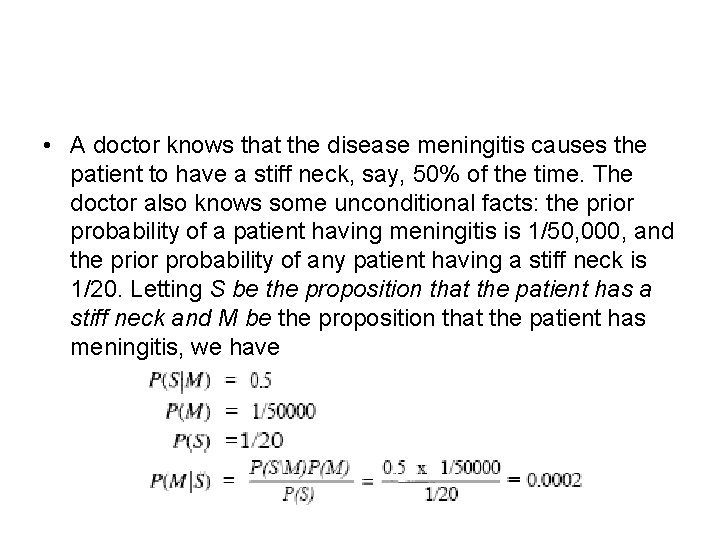• A doctor knows that the disease meningitis causes the patient to have a stiff neck, say, 50% of the time. The doctor also knows some unconditional facts: the prior probability of a patient having meningitis is 1/50, 000, and the prior probability of any patient having a stiff neck is 1/20. Letting S be the proposition that the patient has a stiff neck and M be the proposition that the patient has meningitis, we have• That is, we expect only one in 5000 patients with a stiff neck to have meningitis. • Notice that even though a stiff neck is strongly indicated by meningitis (probability 0. 5), the probability of meningitis in the patient remains small. • This is because the prior on stiff necks is much higher than that for meningitis.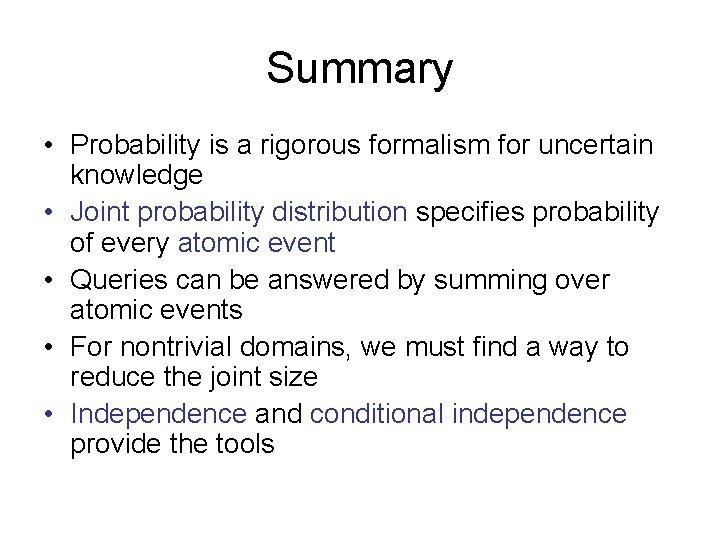Summary • Probability is a rigorous formalism for uncertain knowledge • Joint probability distribution specifies probability of every atomic event • Queries can be answered by summing over atomic events • For nontrivial domains, we must find a way to reduce the joint size • Independence and conditional independence provide the tools